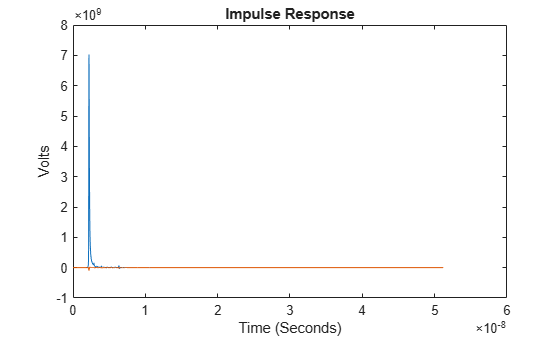# pulse2impulse

Impulse response from pulse response

Since R2020a

## Syntax

``I = pulse2impulse(P,N,dt)``

## Description

example

````I = pulse2impulse(P,N,dt)` converts a pulse response `P` to an impulse response `I`, given the number of samples per symbol `N` and uniform sampling interval `dt`.The function computes the step response from the pulse response. The derivative of the pulse response is the impulse response.The function calculates the derivative with zero delay and is reasonably accurate upto one half of the Nyquist rate. But at the Nyquist rate, the derivative calculation diverges rapidly back to zero.```

## Examples

collapse all

Load the pulse response column matrix from a file.

`load('PulseResponseReflective100ps.mat'); `

Calculate the sample interval.

`dt = SymbolTime/SamplesPerSymbol;`

Calculate the impulse response.

`I = pulse2impulse(pulse,SamplesPerSymbol,dt );`

Plot the impulse response.

```figure plot(t,I) xlabel('Time (Seconds)') ylabel('Volts') title('Impulse Response')```## Input Arguments

collapse all

Input pulse response, specified as a column matrix. The first column contains the primary pulse response and the subsequent columns (if any) contain the crosstalk pulse responses.

Data Types: `double`

Number of samples per symbol, specified as a positive integer scalar.

Data Types: `double`

Uniform timestep of the waveform, specified as a real positive scalar in seconds.

Data Types: `double`

## Output Arguments

collapse all

Converted impulse response, returned as a column matrix. The first column contains the primary impulse response and the subsequent columns (if any) contain the crosstalk impulse responses.

Data Types: `double`

## Version History

Introduced in R2020a